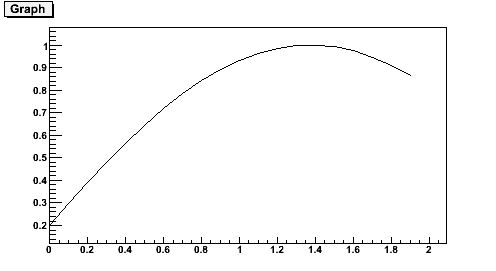# Problem with saving graphs

Hi,

I would like to save the graphs by ROOT, so I tried “Print” and “SaveAs” commands.
However, it creates blank png or pdf files without any error messages
even though it shows the graphs on the screen.
Would you tell me how to save the graphs?

Here is my macro,

{

TCanvas *fCanv;
Int_t *xCanDiv;
Int_t *yCanDiv;
Int_t xCanSize;
Int_t yCanSize;

name = Form(“Protons_”);

name += i;
fCanv[i] = new TCanvas(name.Data(), name.Data(), xCanSize, yCanSize);
fCanv[i]->SetFillColor(10);
fCanv[i]->Divide(xCanDiv[i], yCanDiv[i]);

fCanv[i]->SaveAs( Form( “%s%s.png”, name.Data(), Polarity.Data() ) );
fCanv[i]->Print( Form( “%s%s.pdf”, name.Data(), Polarity.Data() ) );

}

It should work. Try this:

``````
{
c1 = new TCanvas("c1","A Simple Graph Example",200,10,500,300);
const Int_t n = 20;
Double_t x[n], y[n];
for (Int_t i=0;i<n;i++) {
x[i] = 0.1*i;
y[i] = sin(x[i]+0.2);
}
gr = new TGraph(n,x,y);
gr->Draw("ALP");
c1->Print("c1.pdf");
c1->Print("c1.png");
}``````

It gives the attached results.
c1.pdf (12.7 KB)Hi,

I am so sorry to reply so late.
I think the problem is

fCanv[i]->SaveAs( Form( “%s%s.png”, name.Data(), Polarity.Data() ) );
fCanv[i]->Print( Form( “%s%s.pdf”, name.Data(), Polarity.Data() ) );

Form() command and the contents of name.Data(), Polarity.Data() .

Thanks anyway!